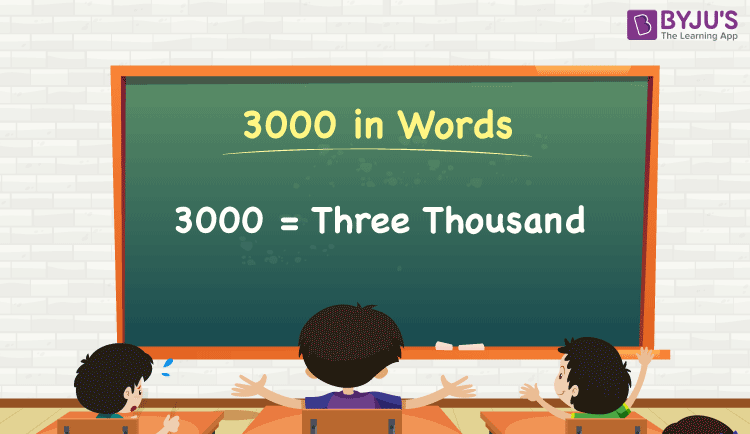# 3000 in Words

We can write 3000 in words as Three thousand. If the number 3000 represents a certain amount of money/the price of a particular thing/total cost of goods, etc., then we express it as Three thousand rupees, and is written as Rs. 3000. Also, note that 3000 is a cardinal number since it denotes a certain quantity.

 3000 in words: Three thousand Three thousand in numerical form: 3000

3000 in English Words

We generally write numbers in words using the English alphabet. Thus, we can read 3000 using the words in English as “Three thousand”.## How to Write 3000 in Words?

We can convert 3000 to words using a place value chart. This can be done as follows.

The number 3000 has 4 digits, so let’s make a chart that shows the place value up to 4 digits.

 Thousands Hundreds Tens Ones 3 0 0 0

Thus, we can write the expanded form as:

3 × Thousand + 0 × Hundred + 0 × Ten + 0 × One

= 3 × 1000 + 0 × 100 + 0 × 10 + 0 × 1

= 3000

= Three thousand

Alternative method:

In the above place value chart, we can observe that the digits in ones, tens, and hundreds are 0s. Hence, we can consider the number at the thousands place, and by combining these terms, we get Three thousand since 3 × 1000 = 3000.

Interesting way of writing 3000 in words:

3 = Three

30 = Thirty

300 = Three hundred

3000 = Three thousand

### Facts About the Number 3000

3000 is a natural number which is the successor of 2999 and the predecessor of 3001.

3000 in words – Three thousand

Is 3000 an odd number? – No

Is 3000 an even number? – Yes

Is 3000 a prime number? – No

Is 3000 a composite number? – Yes

Is 3000 a perfect square number? – No

Is 3000 a perfect cube number? – No

## Frequently Asked Questions on 3000 in Words

Q1

### How do you write 3000 in English?

The spelling of 3000 in English words is Three thousand, which means 3000 in words can be expressed as Three thousand.

Q2

### How do you write 3000 in words on a cheque?

On a cheque, we generally write 3000 in words as Three thousand rupees only.

Q3

### How do you write Rs. 3000 in words?

Rs. 3000 can be written in words as Three thousand rupees.